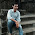Wednesday, 16 September 2015

Bouncing Ball Program in JAVA using Multithreading and AppletBouncing Balls
In the last post we learned what is & how to use Multithreading in JAVA. The next ideal task to learn is how to use multithreading for Animation or how to use Multithreading in Applets. Today we will discuss program of Bouncing Balls in Java by using Multithreading and Applet.  I am going have a button to start the Balls from one point in the Applet window. We need Applet class, AWT class to perform this particular task.

The flow or working of the program is as follows,

1. Compile java program.
2. Execute java program.
3. Click on "Start" Button to start First Ball moving into the frame.
4. Click on "Start" Button again to start Second Ball moving into the frame.

Note: After two clicks the "Start" Button is disabled.

The strategy used in this example is very simple. Each thread is assigned to one Ball. You may modify the given code as per your requirement. Check above Image to understand an instance of execution of the same program. Also following are another Images of same program at different time intervals.

Following is the sample code of the implementation. You can also get it from my GitHub repository.

``````
import java.applet.*;
import java.awt.*;
import java.awt.event.*;
/**
*
* @author YogeshD
*/
/*<applet code="bouncingball/BouncingBall.class" width="500" height="500">
</applet>*/
public class BouncingBall extends Applet implements ActionListener{

/**
* @param args the command line arguments
*/
Button btn;
Ball b1,b2;
int cntr=0;

public void init()
{
btn =new Button("Start");
}
public void paint(Graphics g)
{
try
{
g.setColor(Color.RED);
g.fillOval(b1.x, b1.y,b1.w,b1.h);
g.setColor(Color.GREEN);
g.fillOval(b2.x,b2.y,b2.w,b2.h);

}catch(NullPointerException e){
}
}

public void actionPerformed(ActionEvent ae)
{
if(cntr==0)
{
b1=new Ball(0,0,50,50);
cntr++;
}
else if(cntr==1)
{
b2=new Ball(75,0,50,50);
cntr++;
}
else
{
btn.setEnabled(false);
}
}

class Ball implements Runnable
{
int x,y,w,h,incr_y,incr_x;
Ball(int x,int y,int w,int h)
{
this.x=x;
this.y=y;
this.w=w;
this.h=h;
incr_y=1;
incr_x=1;
t.start();
}
public void run()
{
while(true)
{
y=y+incr_y;
x=x+incr_x;

if((y==getSize().height-h)||(y==0))
incr_y=(-1)*incr_y;
if(x==getSize().width-w||x==0)
incr_x=(-1)*incr_x;

try
{
t.sleep(10);
}
catch(InterruptedException e)
{

}
repaint();
}
}
}
}```
```

==>Posted By Yogesh B. Desai

1.1.